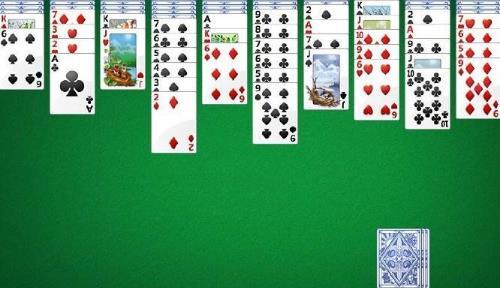• 在线客服

•扫描二维码
下载博学谷APP

•扫描二维码
关注博学谷微信公众号

# 原创 Java课程设计蜘蛛纸牌 实战项目技巧总结

相信大家对蜘蛛纸牌这个游戏本身并不陌生，它是一款桌面休闲益智类游戏。本文小编将来介绍Java课程设计&mdash;—蜘蛛纸牌。本文主要总结了该项目的简介、采用技术、亮点和主要类及关键代码。一、蜘蛛纸牌项目简介。

该课程设计内容主要是模拟实现蜘蛛纸牌游戏，《蜘蛛纸牌》是由Oberon Games开发的一款休闲益智类游戏，该游戏已由Microsoft于2010年发行。同时该游戏也是Windows ME及后续版本所自带的小游戏，游戏目标是以最少的移动次数将牌面中的十叠牌以及待发的五副，共计八副牌整理移除。当所有牌都被移除整理之后，游戏获胜。

二、蜘蛛纸牌项目采用技术。

JAVA

GUI

三、蜘蛛纸牌项目亮点。

1、实现了简单、正常、复杂三种不同难度的玩法

2、可以显示可行性操作

四、蜘蛛纸牌项目主要类及关键代码。

//方法：显示可移动的操作

public void showEnableOperator(){

int x = 0;

out: while (true){

Point point = null;

PKCard card = null;

do{

if (point != null){

n++;

}

point = this.getLastCardLocation(n);

while (point == null){

point = this.getLastCardLocation(++n);

if (n == 10) n = 0;

x++;

if (x == 10) break out;

}

card = (PKCard) this.table.get(point);

}

while (!card.isCardCanMove());

while (this.getPreviousCard(card) != null

&& this.getPreviousCard(card).isCardCanMove()){

card = this.getPreviousCard(card);

}

if (a == 10){

a = 0;

}

for (; a < 10; a++){

if (a != n){

Point p = null;

PKCard c = null;

do{

if (p != null){

a++;

}

p = this.getLastCardLocation(a);

int z = 0;

while (p == null){

p = this.getLastCardLocation(++a);

if (a == 10) a = 0;

if (a == n) a++;

z++;

if (z == 10) break out;

}

c = (PKCard) this.table.get(p);

}

while (!c.isCardCanMove());

if (c.getCardValue() == card.getCardValue() + 1){

card.flashCard(card);

try{

}

catch (InterruptedException e){

e.printStackTrace();

}

c.flashCard(c);

a++;

if (a == 10){

n++;

}

break out;

}

}

}

n++;

if (n == 10){

n = 0;

}

x++;

if (x == 10){

break out;

}

}

}

以上就是Java课程设计蜘蛛纸牌的实战项目技巧总结。大家都明白了吗？如果懂了，就赶紧自己动手编写一个吧！

### 热门文章

• 前端是什么
• 前端开发的工作职责
• 前端开发需要会什么？先掌握这三大核心关键技术
• 前端开发的工作方向有哪些？
• 简历加分-4步写出HR想要的简历
• 程序员如何突击面试？两大招带你拿下面试官
• 程序员面试技巧
• 架构师的厉害之处竟然是这……
• 架构师书籍推荐
• 懂了这些，才能成为架构师
• 查看更多